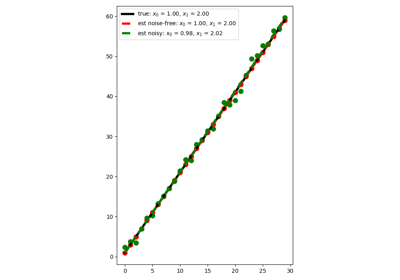# pylops.optimization.cls_sparsity.IRLS#

class pylops.optimization.cls_sparsity.IRLS(Op, callbacks=None)[source]#

Iteratively reweighted least squares.

Solve an optimization problem with $$L_1$$ cost function (data IRLS) or $$L_1$$ regularization term (model IRLS) given the operator Op and data y.

In the data IRLS, the cost function is minimized by iteratively solving a weighted least squares problem with the weight at iteration $$i$$ being based on the data residual at iteration $$i-1$$. This IRLS solver is robust to outliers since the L1 norm given less weight to large residuals than L2 norm does.

Similarly in the model IRLS, the weight at at iteration $$i$$ is based on the model at iteration $$i-1$$. This IRLS solver inverts for a sparse model vector.

Parameters
Oppylops.LinearOperator

Operator to invert

Raises
NotImplementedError

If kind is different from model or data

Notes

Data IRLS solves the following optimization problem for the operator $$\mathbf{Op}$$ and the data $$\mathbf{y}$$:

$J = \|\mathbf{y} - \mathbf{Op}\,\mathbf{x}\|_1$

by a set of outer iterations which require to repeatedly solve a weighted least squares problem of the form:

$\DeclareMathOperator*{\argmin}{arg\,min} \mathbf{x}^{(i+1)} = \argmin_\mathbf{x} \|\mathbf{y} - \mathbf{Op}\,\mathbf{x}\|_{2, \mathbf{R}^{(i)}}^2 + \epsilon_\mathbf{I}^2 \|\mathbf{x}\|_2^2$

where $$\mathbf{R}^{(i)}$$ is a diagonal weight matrix whose diagonal elements at iteration $$i$$ are equal to the absolute inverses of the residual vector $$\mathbf{r}^{(i)} = \mathbf{y} - \mathbf{Op}\,\mathbf{x}^{(i)}$$ at iteration $$i$$. More specifically the $$j$$-th element of the diagonal of $$\mathbf{R}^{(i)}$$ is

$R^{(i)}_{j,j} = \frac{1}{\left| r^{(i)}_j \right| + \epsilon_\mathbf{R}}$

or

$R^{(i)}_{j,j} = \frac{1}{\max\{\left|r^{(i)}_j\right|, \epsilon_\mathbf{R}\}}$

depending on the choice threshR. In either case, $$\epsilon_\mathbf{R}$$ is the user-defined stabilization/thresholding factor .

Similarly model IRLS solves the following optimization problem for the operator $$\mathbf{Op}$$ and the data $$\mathbf{y}$$:

$J = \|\mathbf{x}\|_1 \quad \text{subject to} \quad \mathbf{y} = \mathbf{Op}\,\mathbf{x}$

by a set of outer iterations which require to repeatedly solve a weighted least squares problem of the form :

$\mathbf{x}^{(i+1)} = \operatorname*{arg\,min}_\mathbf{x} \|\mathbf{x}\|_{2, \mathbf{R}^{(i)}}^2 \quad \text{subject to} \quad \mathbf{y} = \mathbf{Op}\,\mathbf{x}$

where $$\mathbf{R}^{(i)}$$ is a diagonal weight matrix whose diagonal elements at iteration $$i$$ are equal to the absolutes of the model vector $$\mathbf{x}^{(i)}$$ at iteration $$i$$. More specifically the $$j$$-th element of the diagonal of $$\mathbf{R}^{(i)}$$ is

$R^{(i)}_{j,j} = \left|x^{(i)}_j\right|.$
1

https://en.wikipedia.org/wiki/Iteratively_reweighted_least_squares

2

Chartrand, R., and Yin, W. “Iteratively reweighted algorithms for compressive sensing”, IEEE. 2008.

Methods

 __init__(Op[, callbacks]) callback(x, *args, **kwargs) Callback routine finalize([show]) Finalize solver run(x[, nouter, show, itershow]) Run solver setup(y[, nouter, threshR, epsR, epsI, ...]) Setup solver solve(y[, x0, nouter, threshR, epsR, epsI, ...]) Run entire solver step(x[, show]) Run one step of solver

## Examples using pylops.optimization.cls_sparsity.IRLS#Linear Regression

Linear Regression